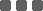## Close price vs Adjusted Close

#### Economy & Market

For the Adjusted Close in Yahoo Finance, I know that it adjusts the Closing price in order to smooth out the effect of stock dividends, stock split, etc. However, I don't find any documentation how Yahoo comes up with those adjusted value. I am curious how the Adjusted Close price is calculated.
Also, am I correct that the concept of "Adjusted Close" is applicable to stock market only?

The adjusted data on Yahoo Finance follows the Center for Research in Security Prices (CRSP) standards.

Here's an example from their article for calculating the split and dividend multipliers.

## Multipliers

Split multipliers are determined by the split ratio.

For example:

• In a 2 for 1 split, the pre-split data is multiplied by 0.5.
• In a 4 for 1 split, the pre-split data is multiplied by 0.25.
• In a 1 for 5 reverse split, the pre-split data is multiplied by 5.

Dividend multipliers are calculated based on dividend as a percentage of the price, primarily to avoid negative historical pricing.

For example:

• If a \$0.08 cash dividend is distributed on Feb 19 (ex- date), and the Feb 18 closing price is \$24.96, the pre-dividend data is multiplied by (1-0.08/24.96) = 0.9968.
• If a \$2.40 cash dividend is distributed on May 12 (ex- date), and the May 11 closing price is \$16.51, the pre-dividend data is multiplied by (1-2.40/16.51) = 0.8546.
• If a \$1.25 cash dividend is distributed on Jan 25 (ex- date), and the Jan 24 closing price is \$51.20, the pre-dividend data is multiplied by (1-1.25/51.20) = 0.9756.

## Sample calculation

Here's how split and dividend multipliers are calculated and applied to determine adjusted close prices.

The first table shows historical prices and the dates of a split and a dividend. The second table shows the calculations.

The multipliers we'll use are derived from the split and dividend:

• Split Multiplier = 0.5
• Dividend Multiplier = 1 - (0.08/24.96) = 0.9968

Using these split and dividend multipliers, adjusted close prices are calculated for dates prior to the split and prior to the dividend ex-date.

• The close price for 2/16 and 2/17 are adjusted for both the split on 2/18 and the ex-dividend date, 2/21.
• The close price for 2/18 through 2/20 are adjusted for the ex-dividend date, 2/21.
• The close price for 2/18 through 2/20 don't need adjustment for the split that occurred before close on 2/18.
• The close price for 2/21 and 2/22 aren't adjusted because they're after the split and ex-dividend dates.
Date Close, Dividend, or Split
2/16 Close = 46.99
2/17 Close = 48.30
2/18 Split = 2:1
Close = 24.96
2/19 Close = 24.91
2/20 Close = 24.95
2/21 Dividend = 0.08 (ex-date)
Close = 24.53
2/22 Close = 24.54

2/16 0.5 * 0.9968 * 46.99 = 23.42
2/17 0.5 * 0.9968 * 48.30 = 24.07
2/18 0.9968 * 24.96 = 24.88
2/19 0.9968 * 24.91 = 24.83
2/20 0.9968 * 24.95 = 24.87
2/21 24.53
2/22 24.54

### Categories

Hot Topic

24 0 1 2023-02-03

Request for parameter optimization function

70 1 1 2023-01-21

187 4 6 2023-01-12

Guideline to call App function in strategy script

155 0 5 2023-01-04

100 2 0 2023-01-03

Guideline to setup OTP Authenticator

143 0 0 2022-12-26

The Opportunities of a Grid Trading System

271 0 1 2022-12-18

3 Common Order Types You Should Know

160 1 2 2022-12-13

Guide to publish your app on App Store

233 4 6 2022-12-02